Anúncio

# GNNs meet RL.pdf

25 de Mar de 2023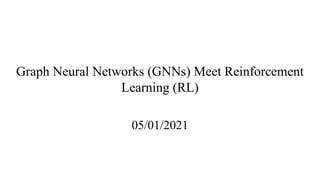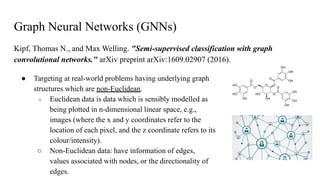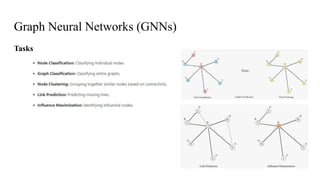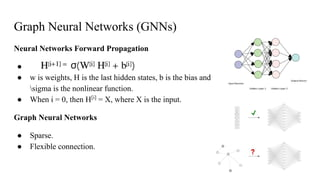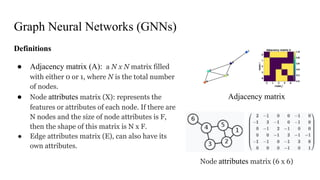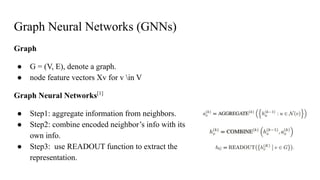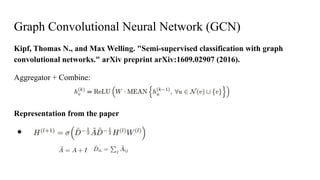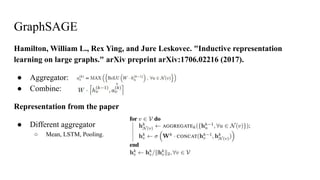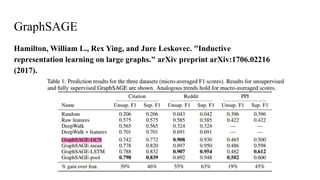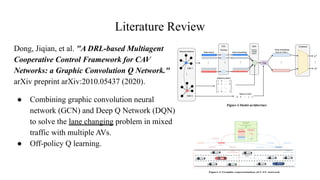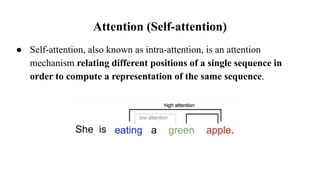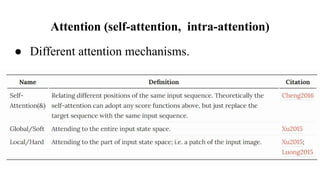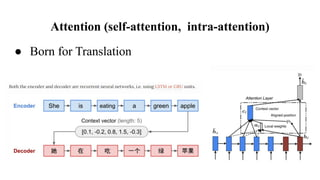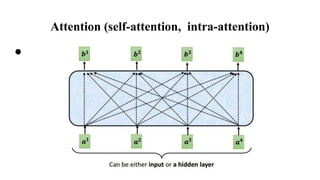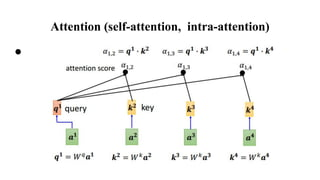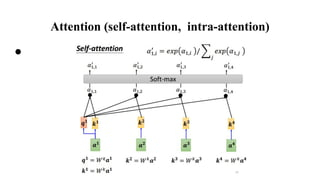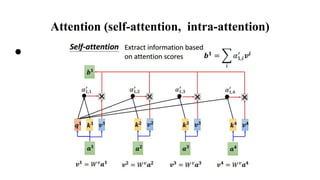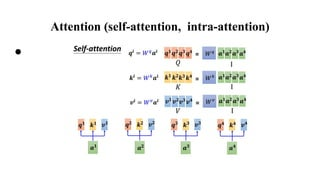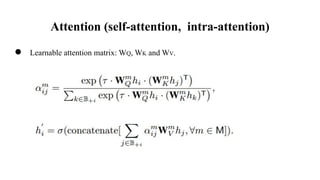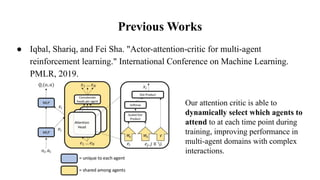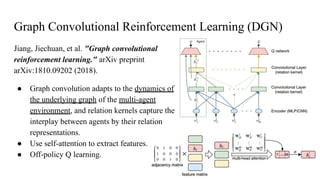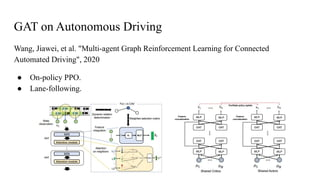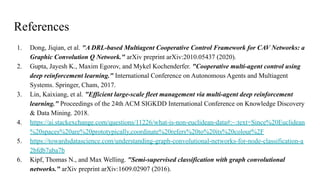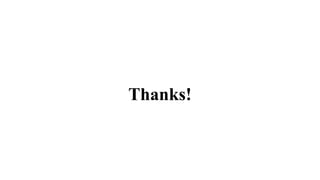1 de 24
Anúncio

### GNNs meet RL.pdf

1. Graph Neural Networks (GNNs) Meet Reinforcement Learning (RL) 05/01/2021
2. Graph Neural Networks (GNNs) Kipf, Thomas N., and Max Welling. "Semi-supervised classification with graph convolutional networks." arXiv preprint arXiv:1609.02907 (2016). ● Targeting at real-world problems having underlying graph structures which are non-Euclidean. ○ Euclidean data is data which is sensibly modelled as being plotted in n-dimensional linear space, e.g., images (where the x and y coordinates refer to the location of each pixel, and the z coordinate refers to its colour/intensity). ○ Non-Euclidean data: have information of edges, values associated with nodes, or the directionality of edges.
3. Graph Neural Networks (GNNs) Tasks
4. Graph Neural Networks (GNNs) Neural Networks Forward Propagation ● ● w is weights, H is the last hidden states, b is the bias and sigma is the nonlinear function. ● When i = 0, then H[i] = X, where X is the input. Graph Neural Networks ● Sparse. ● Flexible connection.
5. Graph Neural Networks (GNNs) Definitions ● Adjacency matrix (A): a N x N matrix filled with either 0 or 1, where N is the total number of nodes. ● Node attributes matrix (X): represents the features or attributes of each node. If there are N nodes and the size of node attributes is F, then the shape of this matrix is N x F. ● Edge attributes matrix (E), can also have its own attributes. Adjacency matrix Node attributes matrix (6 x 6)
6. Graph Neural Networks (GNNs) Graph ● G = (V, E), denote a graph. ● node feature vectors Xv for v in V Graph Neural Networks ● Step1: aggregate information from neighbors. ● Step2: combine encoded neighbor’s info with its own info. ● Step3: use READOUT function to extract the representation.
7. Graph Convolutional Neural Network (GCN) Kipf, Thomas N., and Max Welling. "Semi-supervised classification with graph convolutional networks." arXiv preprint arXiv:1609.02907 (2016). Aggregator + Combine: Representation from the paper ●
8. GraphSAGE Hamilton, William L., Rex Ying, and Jure Leskovec. "Inductive representation learning on large graphs." arXiv preprint arXiv:1706.02216 (2017). ● Aggregator: ● Combine: Representation from the paper ● Different aggregator ○ Mean, LSTM, Pooling.
9. GraphSAGE Hamilton, William L., Rex Ying, and Jure Leskovec. "Inductive representation learning on large graphs." arXiv preprint arXiv:1706.02216 (2017).
10. Literature Review Dong, Jiqian, et al. "A DRL-based Multiagent Cooperative Control Framework for CAV Networks: a Graphic Convolution Q Network." arXiv preprint arXiv:2010.05437 (2020). ● Combining graphic convolution neural network (GCN) and Deep Q Network (DQN) to solve the lane changing problem in mixed traffic with multiple AVs. ● Off-policy Q learning.
11. Attention (Self-attention) ● Self-attention, also known as intra-attention, is an attention mechanism relating different positions of a single sequence in order to compute a representation of the same sequence.
12. Attention (self-attention, intra-attention) ● Different attention mechanisms.
13. Attention (self-attention, intra-attention) ● Born for Translation
14. Attention (self-attention, intra-attention) ●
15. Attention (self-attention, intra-attention) ●
16. Attention (self-attention, intra-attention) ●
17. Attention (self-attention, intra-attention) ●
18. Attention (self-attention, intra-attention) ●
19. Attention (self-attention, intra-attention) ● Learnable attention matrix: WQ, WK and WV.
20. Previous Works ● Iqbal, Shariq, and Fei Sha. "Actor-attention-critic for multi-agent reinforcement learning." International Conference on Machine Learning. PMLR, 2019. Our attention critic is able to dynamically select which agents to attend to at each time point during training, improving performance in multi-agent domains with complex interactions.
21. Graph Convolutional Reinforcement Learning (DGN) Jiang, Jiechuan, et al. "Graph convolutional reinforcement learning." arXiv preprint arXiv:1810.09202 (2018). ● Graph convolution adapts to the dynamics of the underlying graph of the multi-agent environment, and relation kernels capture the interplay between agents by their relation representations. ● Use self-attention to extract features. ● Off-policy Q learning.
22. GAT on Autonomous Driving Wang, Jiawei, et al. "Multi-agent Graph Reinforcement Learning for Connected Automated Driving", 2020 ● On-policy PPO. ● Lane-following.
23. References 1. Dong, Jiqian, et al. "A DRL-based Multiagent Cooperative Control Framework for CAV Networks: a Graphic Convolution Q Network." arXiv preprint arXiv:2010.05437 (2020). 2. Gupta, Jayesh K., Maxim Egorov, and Mykel Kochenderfer. "Cooperative multi-agent control using deep reinforcement learning." International Conference on Autonomous Agents and Multiagent Systems. Springer, Cham, 2017. 3. Lin, Kaixiang, et al. "Efficient large-scale fleet management via multi-agent deep reinforcement learning." Proceedings of the 24th ACM SIGKDD International Conference on Knowledge Discovery & Data Mining. 2018. 4. https://ai.stackexchange.com/questions/11226/what-is-non-euclidean-data#:~:text=Since%20Euclidean %20spaces%20are%20prototypically,coordinate%20refers%20to%20its%20colour%2F 5. https://towardsdatascience.com/understanding-graph-convolutional-networks-for-node-classification-a 2bfdb7aba7b 6. Kipf, Thomas N., and Max Welling. "Semi-supervised classification with graph convolutional networks." arXiv preprint arXiv:1609.02907 (2016).
24. Thanks!
Anúncio